# Differentiation¶

## Numerical derivatives (diff, diffs)¶

mpmath.diff(ctx, f, x, n=1, **options)

Numerically computes the derivative of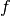,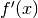, or generally for an integer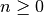, the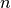-th derivative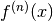. A few basic examples are:

>>> from mpmath import *
>>> mp.dps = 15; mp.pretty = True
>>> diff(lambda x: x**2 + x, 1.0)
3.0
>>> diff(lambda x: x**2 + x, 1.0, 2)
2.0
>>> diff(lambda x: x**2 + x, 1.0, 3)
0.0
>>> nprint([diff(exp, 3, n) for n in range(5)])   # exp'(x) = exp(x)
[20.0855, 20.0855, 20.0855, 20.0855, 20.0855]


Even more generally, given a tuple of arguments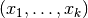and order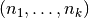, the partial derivative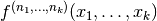is evaluated. For example:

>>> diff(lambda x,y: 3*x*y + 2*y - x, (0.25, 0.5), (0,1))
2.75
>>> diff(lambda x,y: 3*x*y + 2*y - x, (0.25, 0.5), (1,1))
3.0


Options

The following optional keyword arguments are recognized:

method
Supported methods are 'step' or 'quad': derivatives may be computed using either a finite difference with a small step size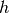(default), or numerical quadrature.
direction
Direction of finite difference: can be -1 for a left difference, 0 for a central difference (default), or +1 for a right difference; more generally can be any complex number.
Extra precision forused to account for the function’s sensitivity to perturbations (default = 10).
relative
Chooserelative to the magnitude of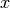, rather than an absolute value; useful for large or tiny(default = False).
h
As an alternative to addprec and relative, manually select the step size.
singular
If True, evaluation exactly at the pointis avoided; this is useful for differentiating functions with removable singularities. Default = False.
Radius of integration contour (with method = 'quad'). Default = 0.25. A larger radius typically is faster and more accurate, but it must be chosen so thathas no singularities within the radius from the evaluation point.

A finite difference requires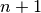function evaluations and must be performed at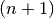times the target precision. Accordingly,must support fast evaluation at high precision.

With integration, a larger number of function evaluations is required, but not much extra precision is required. For high order derivatives, this method may thus be faster if f is very expensive to evaluate at high precision.

Further examples

The direction option is useful for computing left- or right-sided derivatives of nonsmooth functions:

>>> diff(abs, 0, direction=0)
0.0
>>> diff(abs, 0, direction=1)
1.0
>>> diff(abs, 0, direction=-1)
-1.0


More generally, if the direction is nonzero, a right difference is computed where the step size is multiplied by sign(direction). For example, with direction=+j, the derivative from the positive imaginary direction will be computed:

>>> diff(abs, 0, direction=j)
(0.0 - 1.0j)


With integration, the result may have a small imaginary part even even if the result is purely real:

>>> diff(sqrt, 1, method='quad')
(0.5 - 4.59...e-26j)
>>> chop(_)
0.5


Adding precision to obtain an accurate value:

>>> diff(cos, 1e-30)
0.0
>>> diff(cos, 1e-30, h=0.0001)
-9.99999998328279e-31
-1.0e-30

mpmath.diffs(ctx, f, x, n=None, **options)

Returns a generator that yields the sequence of derivatives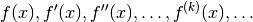With method='step', diffs() uses only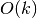function evaluations to generate the first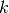derivatives, rather than the roughly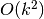evaluations required if one calls diff()separate times.

With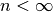, the generator stops as soon as the-th derivative has been generated. If the exact number of needed derivatives is known in advance, this is further slightly more efficient.

Options are the same as for diff().

Examples

>>> from mpmath import *
>>> mp.dps = 15
>>> nprint(list(diffs(cos, 1, 5)))
[0.540302, -0.841471, -0.540302, 0.841471, 0.540302, -0.841471]
>>> for i, d in zip(range(6), diffs(cos, 1)):
...     print("%s %s" % (i, d))
...
0 0.54030230586814
1 -0.841470984807897
2 -0.54030230586814
3 0.841470984807897
4 0.54030230586814
5 -0.841470984807897


## Composition of derivatives (diffs_prod, diffs_exp)¶

mpmath.diffs_prod(ctx, factors)

Given a list of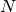iterables or generators yielding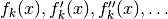for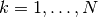, generatewhere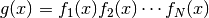.

At high precision and for large orders, this is typically more efficient than numerical differentiation if the derivatives of each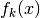admit direct computation.

Note: This function does not increase the working precision internally, so guard digits may have to be added externally for full accuracy.

Examples

>>> from mpmath import *
>>> mp.dps = 15; mp.pretty = True
>>> f = lambda x: exp(x)*cos(x)*sin(x)
>>> u = diffs(f, 1)
>>> v = mp.diffs_prod([diffs(exp,1), diffs(cos,1), diffs(sin,1)])
>>> next(u); next(v)
1.23586333600241
1.23586333600241
>>> next(u); next(v)
0.104658952245596
0.104658952245596
>>> next(u); next(v)
-5.96999877552086
-5.96999877552086
>>> next(u); next(v)
-12.4632923122697
-12.4632923122697

mpmath.diffs_exp(ctx, fdiffs)

Given an iterable or generator yielding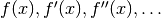generatewhere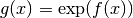.

At high precision and for large orders, this is typically more efficient than numerical differentiation if the derivatives of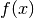admit direct computation.

Note: This function does not increase the working precision internally, so guard digits may have to be added externally for full accuracy.

Examples

The derivatives of the gamma function can be computed using logarithmic differentiation:

>>> from mpmath import *
>>> mp.dps = 15; mp.pretty = True
>>>
>>> def diffs_loggamma(x):
...     yield loggamma(x)
...     i = 0
...     while 1:
...         yield psi(i,x)
...         i += 1
...
>>> u = diffs_exp(diffs_loggamma(3))
>>> v = diffs(gamma, 3)
>>> next(u); next(v)
2.0
2.0
>>> next(u); next(v)
1.84556867019693
1.84556867019693
>>> next(u); next(v)
2.49292999190269
2.49292999190269
>>> next(u); next(v)
3.44996501352367
3.44996501352367


## Fractional derivatives / differintegration (differint)¶

mpmath.differint(ctx, f, x, n=1, x0=0)

Calculates the Riemann-Liouville differintegral, or fractional derivative, defined by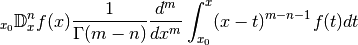whereis a given (presumably well-behaved) function,is the evaluation point,is the order, and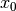is the reference point of integration (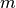is an arbitrary parameter selected automatically).

With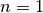, this is just the standard derivative; with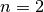, the second derivative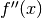, etc. With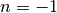, it gives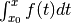, with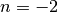it gives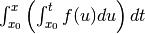, etc.

Asis permitted to be any number, this operator generalizes iterated differentiation and iterated integration to a single operator with a continuous order parameter.

Examples

There is an exact formula for the fractional derivative of a monomial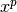, which may be used as a reference. For example, the following gives a half-derivative (order 0.5):

>>> from mpmath import *
>>> mp.dps = 15; mp.pretty = True
>>> x = mpf(3); p = 2; n = 0.5
>>> differint(lambda t: t**p, x, n)
7.81764019044672
>>> gamma(p+1)/gamma(p-n+1) * x**(p-n)
7.81764019044672


Another useful test function is the exponential function, whose integration / differentiation formula easy generalizes to arbitrary order. Here we first compute a third derivative, and then a triply nested integral. (The reference pointis set to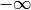to avoid nonzero endpoint terms.):

>>> differint(lambda x: exp(pi*x), -1.5, 3)
0.278538406900792
>>> exp(pi*-1.5) * pi**3
0.278538406900792
>>> differint(lambda x: exp(pi*x), 3.5, -3, -inf)
1922.50563031149
>>> exp(pi*3.5) / pi**3
1922.50563031149


However, for noninteger, the differentiation formula for the exponential function must be modified to give the same result as the Riemann-Liouville differintegral:

>>> x = mpf(3.5)
>>> c = pi
>>> n = 1+2*j
>>> differint(lambda x: exp(c*x), x, n)
(-123295.005390743 + 140955.117867654j)
>>> x**(-n) * exp(c)**x * (x*c)**n * gammainc(-n, 0, x*c) / gamma(-n)
(-123295.005390743 + 140955.117867654j)